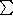CGAL::Hyperplane_d<Kernel>

Definition

An instance of data type Hyperplane_d is an oriented hyperplane in d - dimensional space. A hyperplane h is represented by coefficients (c0,c1,...,cd) of type RT. At least one of c0 to c d - 1 must be non-zero. The plane equation is0i < d ci xi + cd = 0, where x0 to xd-1 are Cartesian point coordinates. For a particular x the sign of0i < d ci xi + cd determines the position of a point x with respect to the hyperplane (on the hyperplane, on the negative side, or on the positive side).

There are two equality predicates for hyperplanes. The (weak) equality predicate (weak_equality) declares two hyperplanes equal if they consist of the same set of points, the strong equality predicate (operator==) requires in addition that the negative halfspaces agree. In other words, two hyperplanes are strongly equal if their coefficient vectors are positive multiples of each other and they are (weakly) equal if their coefficient vectors are multiples of each other.

Types

 Hyperplane_d::LA the linear algebra layer. Hyperplane_d::Coefficient_const_iterator a read-only iterator for the coefficients.

Creation

Hyperplane_d<Kernel> h;
introduces a variable h of type Hyperplane_d<Kernel>.

template <class InputIterator>
Hyperplane_d<Kernel> h ( int d, InputIterator first, InputIterator last, RT D);
introduces a variable h of type Hyperplane_d<Kernel> initialized to the hyperplane with coefficients set [first,last) and D.
 Precondition: size [first,last) == d.
 Requirement: The value type of InputIterator is RT.

template <class InputIterator>
Hyperplane_d<Kernel> h ( int d, InputIterator first, InputIterator last);
introduces a variable h of type Hyperplane_d<Kernel> initialized to the hyperplane with coefficients set [first,last).
 Precondition: size [first,last) == d+1.
 Requirement: The value type of InputIterator is RT.

template <class ForwardIterator>
Hyperplane_d<Kernel> h ( ForwardIterator first, ForwardIterator last, Point_d<Kernel> o, Oriented_side side = ON_ORIENTED_BOUNDARY);
constructs some hyperplane that passes through the points in set [first,last). If side is ON_POSITIVE_SIDE or ON_NEGATIVE_SIDE then o is on that side of the constructed hyperplane.
 Precondition: A hyperplane with the stated properties must exist.
 Requirement: The value type of ForwardIterator is Point_d.

Hyperplane_d<Kernel> h ( Point_d<Kernel> p, Direction_d<Kernel> dir);
constructs the hyperplane with normal direction dir that passes through p. The direction dir points into the positive side.
 Precondition: p.dimension()==dir.dimension() and dir is not degenerate.

Hyperplane_d<Kernel> h ( RT a, RT b, RT c);
introduces a variable h of type Hyperplane_d<Kernel> in 2-dimensional space with equation ax+by+c=0.

Hyperplane_d<Kernel> h ( RT a, RT b, RT c, RT d);
introduces a variable h of type Hyperplane_d<Kernel> in 3-dimensional space with equation ax+by+cz+d=0.

Operations

int h.dimension () returns the dimension of h.

RT h [ int i ] returns the i-th coefficient of h.
 Precondition: 0id.

RT h.coefficient ( int i) returns the i-th coefficient of h.
 Precondition: 0id.

Coefficient_const_iterator h.coefficients_begin () returns an iterator pointing to the first coefficient.

Coefficient_const_iterator h.coefficients_end () returns an iterator pointing beyond the last coefficient.

Vector_d<Kernel> h.orthogonal_vector () returns the orthogonal vector of h. It points from the negative halfspace into the positive halfspace and its homogeneous coordinates are (c0, ..., cd - 1,1).

Direction_d<Kernel> h.orthogonal_direction () returns the orthogonal direction of h. It points from the negative halfspace into the positive halfspace.

Oriented_side h.oriented_side ( Point_d<Kernel> p)
returns the side of the hyperplane h containing p.
 Precondition: h.dimension() == p.dimension().

bool h.has_on ( Point_d<Kernel> p) returns true iff point p lies on the hyperplane h.
 Precondition: h.dimension() == p.dimension().

bool h.has_on_boundary ( Point_d<Kernel> p)
returns true iff point p lies on the boundary of hyperplane h.
 Precondition: h.dimension() == p.dimension().

bool h.has_on_positive_side ( Point_d<Kernel> p)
returns true iff point p lies on the positive side of hyperplane h.
 Precondition: h.dimension() == p.dimension().

bool h.has_on_negative_side ( Point_d<Kernel> p)
returns true iff point p lies on the negative side of hyperplane h.
 Precondition: h.dimension() == p.dimension().

Hyperplane_d<Kernel> h.transform ( Aff_transformation_d<Kernel> t)
returns t(h).
 Precondition: h.dimension() == t.dimension().

Non-Member Functions

bool weak_equality ( h1, h2) test for weak equality.
 Precondition: h1.dimension() == h2.dimension().

Implementation

Hyperplanes are implemented by arrays of integers as an item type. All operations like creation, initialization, tests, vector arithmetic, input and output on a hyperplane h take time O(h.dimension()). coordinate access and dimension() take constant time. The space requirement is O(h.dimension()).